Courses

# Analysis Of a Transistor Amplifier Circuit Using H-Parameters Electrical Engineering (EE) Notes | EduRev

## Electronic Devices

Created by: Cstoppers Instructors

## Electrical Engineering (EE) : Analysis Of a Transistor Amplifier Circuit Using H-Parameters Electrical Engineering (EE) Notes | EduRev

The document Analysis Of a Transistor Amplifier Circuit Using H-Parameters Electrical Engineering (EE) Notes | EduRev is a part of the Electrical Engineering (EE) Course Electronic Devices.
All you need of Electrical Engineering (EE) at this link: Electrical Engineering (EE)

ANALYSIS OF A TRANSISTOR AMPLIFIER USING H-PARAMETERS:

To form a transistor amplifier it is only necessary to connect an external load and signal source as indicated in fig. 1 and to bias the transistor properly.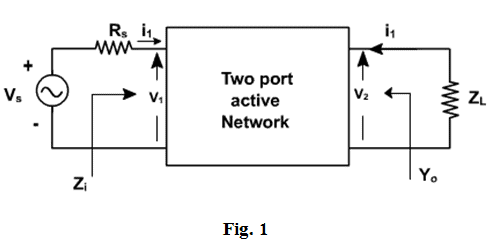Consider the two-port network of CE amplifier. RS is the source resistance and ZL is the load impedance. The h-parameters are assumed to be constant over the operating range. The ac equivalent circuit is shown in fig. 2. (Phasor notations are used assuming sinusoidal voltage input). The quantities of interest are the current gain, input impedance, voltage gain, and output impedance.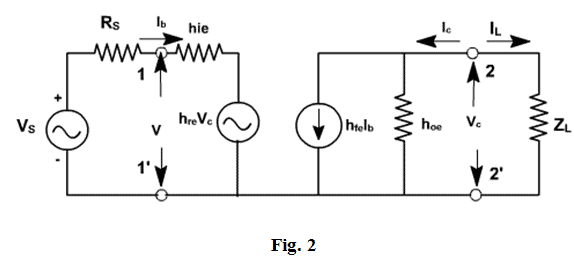Current gain:

For the transistor amplifier stage, Ai is defined as the ratio of output to input currents.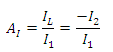1.3.2 Input impedance:

The impedance looking into the amplifier input terminals ( 1,1' ) is the input impedance Zi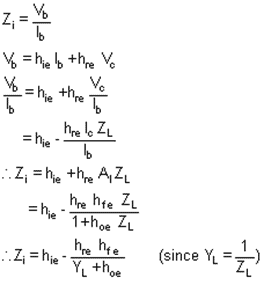Voltage gain:

The ratio of output voltage to input voltage gives the gain of the transistors.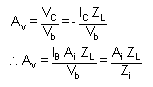It is defined as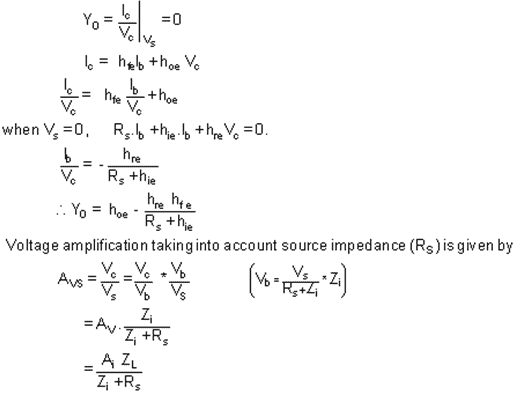Av is the voltage gain for an ideal voltage source (Rv = 0).

Consider input source to be a current source IS in parallel with a resistance RS as shown in fig. 3.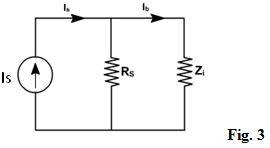In this case, overall current gain AIS is defined as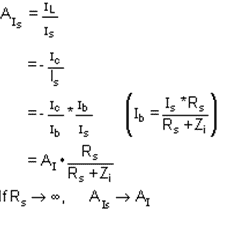h-parameters:
To analyze multistage amplifier the h-parameters of the transistor used are obtained from manufacturer data sheet. The manufacturer data sheet usually provides h-parameter in CE configuration. These parameters may be converted into CC and CB values. For exampl
e fig. 4 hrc in terms of CE parameter can be obtained as follows.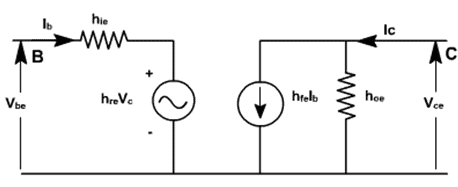Fig. 4

For CE transistor configuaration

Vbe = hie Ib + hre Vce

Ic = hfe Ib + hoe Vce

The circuit can be redrawn like CC transistor configuration as shown in fig. 5.Vbc = hie Ib + hrc Vec

Ic = hfe Ib + hoe Vec

Hybrid model for transistor in three different configurations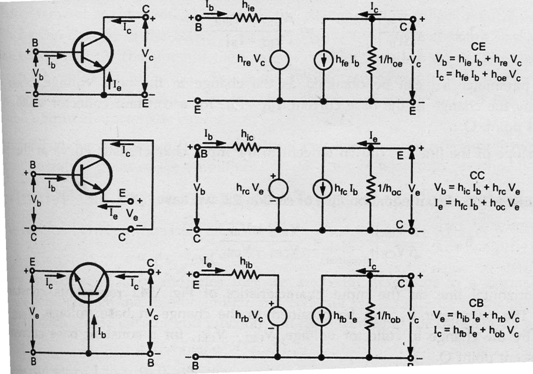Typical h-parameter values for a transistor: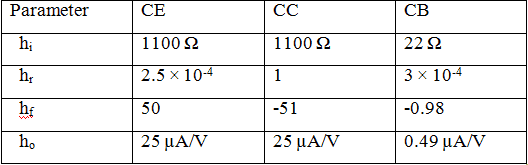Analysis of a Transistor amplifier circuit using h-parameters

A transistor amplifier can be constructed by connecting an external load and signal source and biasing the transistor properly.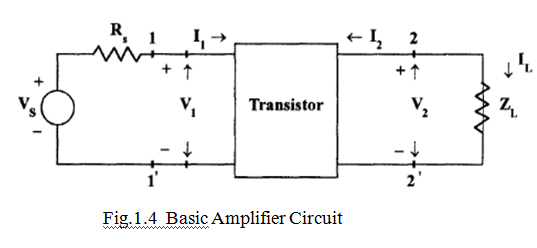The two port network of Fig. 1.4  represents a transistor in any one of its configuration. It is assumed that h-parameters remain constant over the operating range.The input is sinusoidal and I1,V­1,I2 and V2 are phase quantities.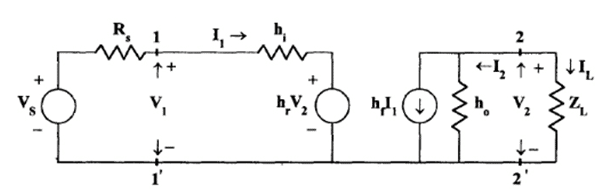Fig. 1.5  Transistor replaced by its Hybrid Model

Current Gain or Current Amplification (Ai)

For transistor amplifier, the current gain Ais defined as the ratio of output current to input current,i.e,

A=IL /I= -I/ I1

From the circuit of Fig 1.5

I2= hI1 + hoV2

Substituting V= ILZ=  -I2ZL

I2= hI1- I2ZL ho

I2 + I2ZL ho = hI1

I2( 1+ ZL ho) = hI1

A=  -I/ I1 = - hf / ( 1+ ZL ho)

Therefore,

Ai = - hf / ( 1+ ZL ho)

Input Impedance (Zi)

In the circuit of Fig 1.5, Ris the signal source resistance .The impedance seen when looking into the amplifier terminals (1,1) is the amplifier input impedance  Zi,

Zi = V1/I1

From the input circuit of Fig   V1 = hI1 + hrV2

Zi = ( hI1 + hrV2) / I1

= h+ hr V2 / I1

Substituting

V2 = -IZ= A1I1ZL

Zi = h+ hr A1I1ZL / I1

= h+ hr A1ZL

Substituting for Ai

Zi = h- hf hr ZL / (1+ hoZL)

= h- hf hr ZL / ZL(1/ZL+ ho)

Zi  = h- hf hr  / (YL + ho)

Voltage Gain or Voltage Gain Amplification Factor(Av)

The ratio of output voltage Vto input voltage Vgive the voltage gain of the transistor i.e,

Av = V2 / V1

Substituting

V2 = -IZ= A1I1ZL

A= A1I1ZL / V1 =  AiZL / Zi

Yo is obtained by setting VS to zero, ZL to infinity and by driving the output terminals from a generator V2. If the current is I2 then Yo= I2/V2 with VS=0 and RL= ∞.

From the circuit of fig 1.5

I2= hI1 + hoV2

Dividing by V2,

I/ V2 = hI1/V2 + ho

With V2= 0, by KVL in input circuit,

RSI1 + hI1 + hrV= 0

(RS + hi) I1 + hrV= 0

Hence,     I/ V2 = -h/ (RS + hi)

= h(-hr/( RS + hi)+ho

Yo= ho- hhr/( RS + hi)

The output admittance is a function of source resistance. If the source impedance is resistive then Yis real.

Voltage Amplification Factor(Avs) is taking into account the resistance (Rs) of the source.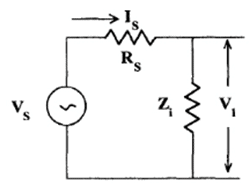Fig. 5.6 Thevenin’s Equivalent Input Circuit

This overall voltage gain Avs is given by

Avs = V/ VS = V2V/ V1VS = Av V1/ VS

From the equivalent input circuit using Thevenin’s equivalent for the source shown in Fig. 5.6,

V1 = VZ/ (Zi + RS)

V/ VS = Z/ ( Zi + RS)

Then,               Avs =  Av Z/ ( Zi + RS)

Substituting     A= AiZL / Zi

Avs =  AiZL / ( Zi + RS)

Avs = AiZL RS / ( Zi + RS) RS

Avs = AisZ/  RS

Current Amplification (Ais) taking into account the source Resistance(RS)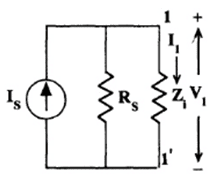Fig. 1.7  Norton’s Equivalent Input Circuit

The modified input circuit using Norton’s equivalent circuit for the calculation of Ais is shown in Fig. 1.7

Overall Current Gain, Ais = -I/ IS = - I2I/I1 IS = Ai I1/IS

From Fig. 1.7                          I1= IS R/ (RS + Zi)

I/ IS = R/ (RS + Zi)

and hence,                   Ais = Ai R/ (RS + Zi)

Operating Power Gain (AP)

The operating power gain Aof the transistor is defined as

A= P2 / P1 = -V2 I2 / V1 I1 = AvA= Ai AiZL/ Zi

A= Ai2(ZL/ Zi)

Small Signal analysis of a transistor amplifier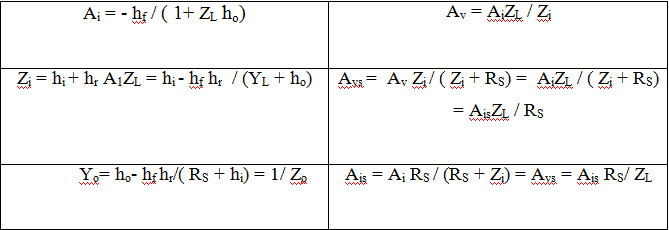Offer running on EduRev: Apply code STAYHOME200 to get INR 200 off on our premium plan EduRev Infinity!

,

,

,

,

,

,

,

,

,

,

,

,

,

,

,

,

,

,

,

,

,

;